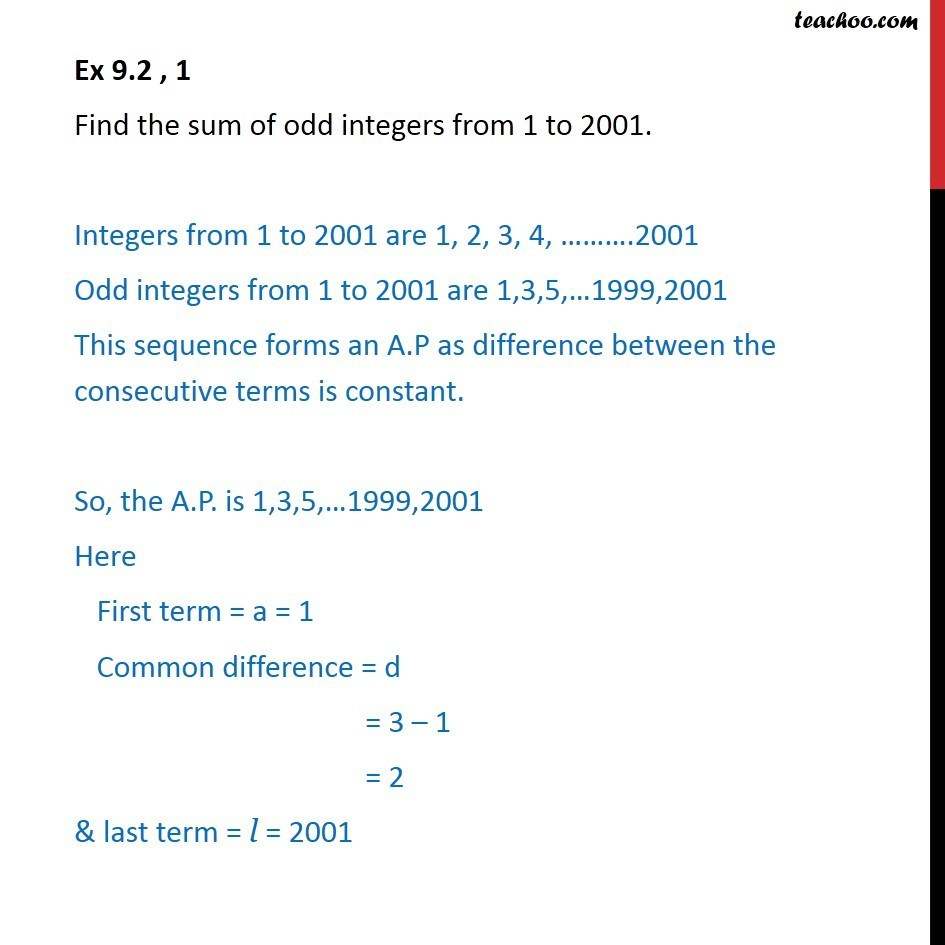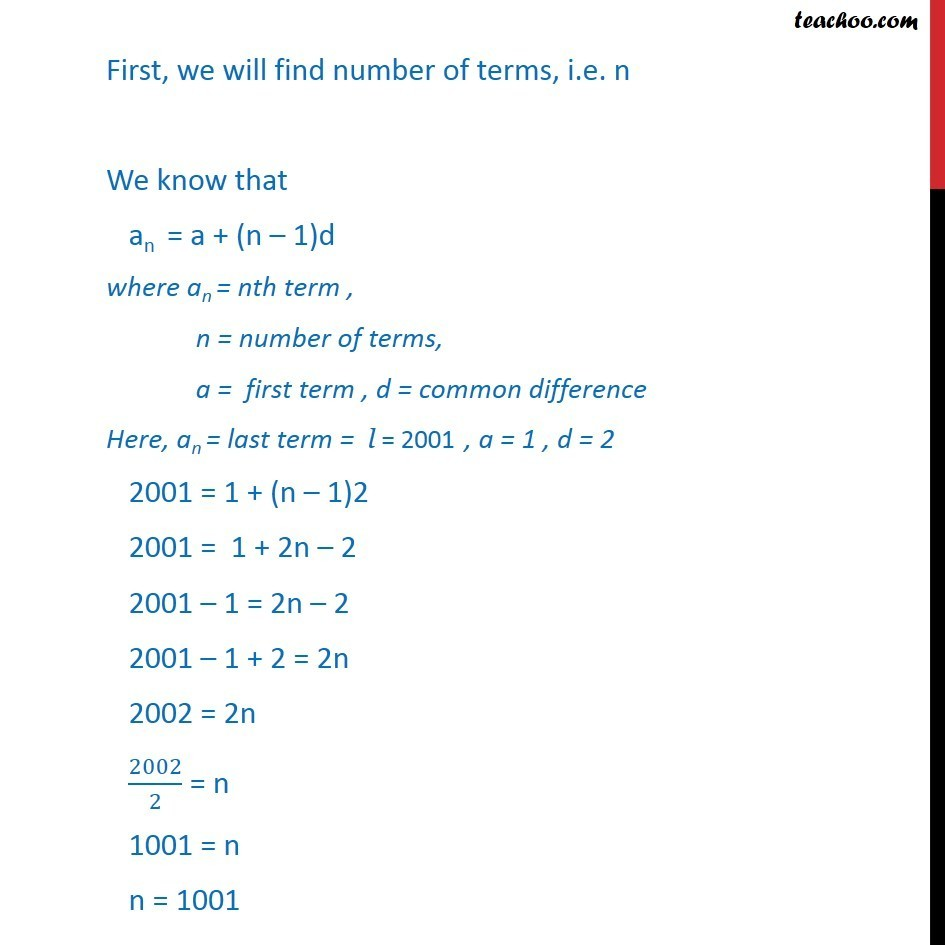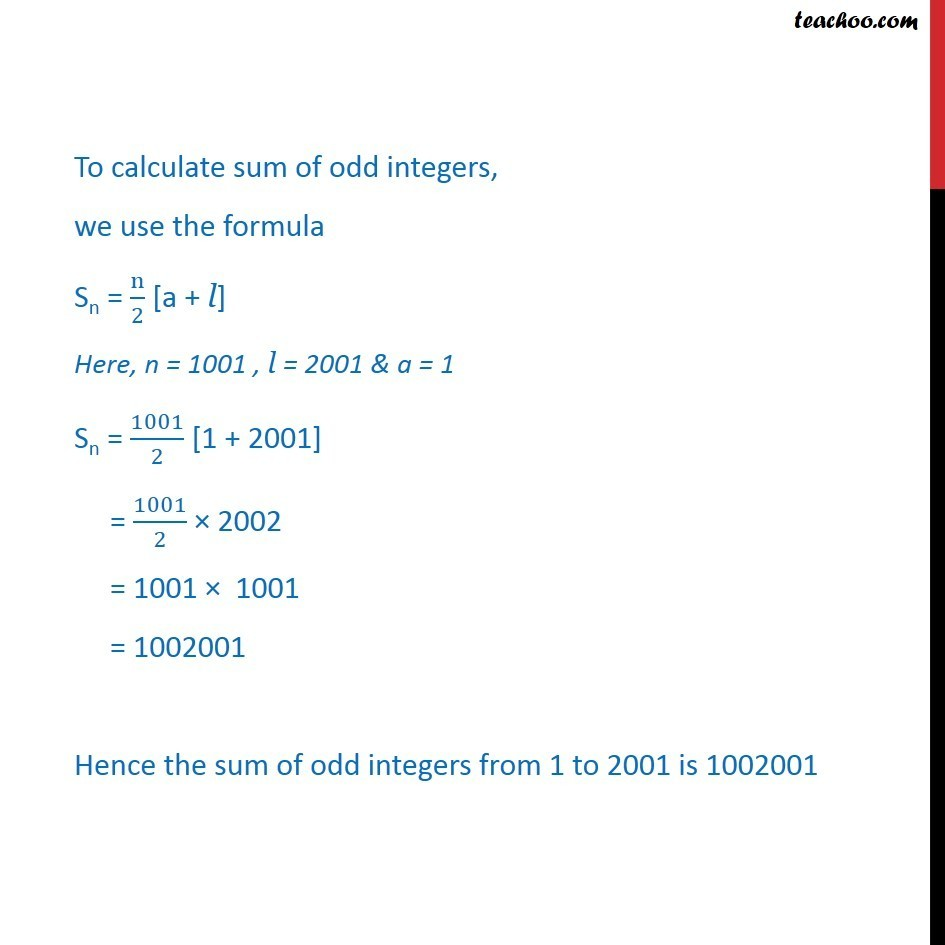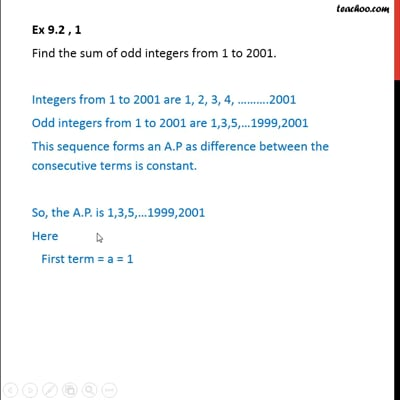Arithmetic Progression (AP): Formulae based

Chapter 9 Class 11 Sequences and Series
Concept wiseThis video is only available for Teachoo black users

Introducing your new favourite teacher - Teachoo Black, at only ₹83 per month

### Transcript

Ex 9.2 , 1 Find the sum of odd integers from 1 to 2001. Integers from 1 to 2001 are 1, 2, 3, 4, .2001 Odd integers from 1 to 2001 are 1,3,5, 1999,2001 This sequence forms an A.P as difference between the consecutive terms is constant. So, the A.P. is 1,3,5, 1999,2001 Here First term = a = 1 Common difference = d = 3 1 = 2 & last term = l = 2001 First, we will find number of terms, i.e. n We know that an = a + (n 1)d where an = nth term , n = number of terms, a = first term , d = common difference Here, an = last term = l = 2001 , a = 1 , d = 2 2001 = 1 + (n 1)2 2001 = 1 + 2n 2 2001 1 = 2n 2 2001 1 + 2 = 2n 2002 = 2n 2002/2 = n 1001 = n n = 1001 To calculate sum of odd integers, we use the formula Sn = n/2 [a + l] Here, n = 1001 , l = 2001 & a = 1 Sn = 1001/2 [1 + 2001] = 1001/2 2002 = 1001 1001 = 1002001 Hence the sum of odd integers from 1 to 2001 is 1002001